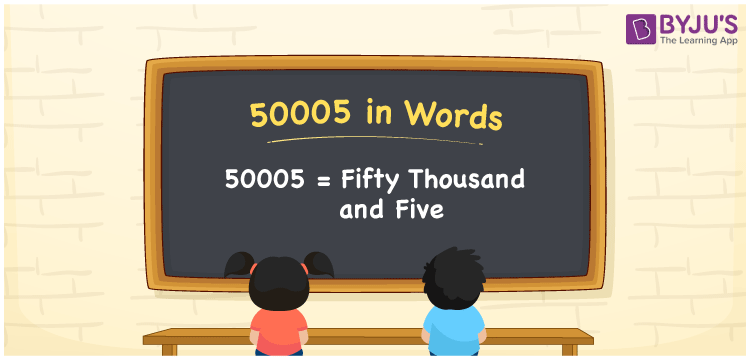# 50005 in Words

50005 in words can be written as Fifty Thousand and Five. The fundamental concepts in Mathematics like counting or count can be learnt efficiently here. If you buy gold worth Rs. 50005, then you can say that “I bought gold ornaments for Fifty Thousand and Five Rupees”. To write numbers in words, the English alphabet is used. The numbers in words concept is explained here in a simple way to improve the conceptual knowledge of students. The 50005 can be read as “Fifty Thousand and Five” in English.

 50005 in words Fifty Thousand and Five Fifty Thousand and Five in Numbers 50005

## 50005 in English Words## How to Write 50005 in Words?

Students can learn about the expanded form and the place value chart of 50005. Five digits are present in the number 50005. With the help of the place value chart given below, students will be able to understand the concepts with ease.

 Ten Thousands Thousands Hundreds Tens Ones 5 0 0 0 5

50005 can be written in expanded form as:

5 x Ten Thousand + 0 x Thousand + 0 × Hundred + 0 × Ten + 5 × One

= 5 x 10000 + 0 x 1000 + 0 × 100 + 0 × 10 + 5 × 1

= 50000 + 5

= 50005

= Fifty Thousand and Five

Hence, 50005 in words is written as Fifty Thousand and Five.

50005 is a natural number that precedes 50006 and succeeds 50004.

50005 in words – Fifty Thousand and Five

Is 50005 an odd number? – Yes

Is 50005 an even number? – No

Is 50005 a perfect square number? – No

Is 50005 a perfect cube number? – No

Is 50005 a prime number? – No

Is 50005 a composite number? – Yes

## Frequently Asked Questions on 50005 in Words

Q1

### How to write 50005 in words?

50005 can be written in words as “Fifty Thousand and Five”.
Q2

### How to write Fifty Thousand and Five in numbers?

Fifty Thousand and Five in numbers can be written as 50005.
Q3

### Is 50005 an odd or even number?

50005 is an odd number as it is not completely divisible by 2.